# Control sit/stand Desk height with a Paper E-Ink Display

Control IKEA Bekant Desk sit/stand height with M5Stack M5Paper using two Relay units and a ToF (time-of-flight sensor VL53L0x) unit.

IntermediateWork in progress4 hours1,497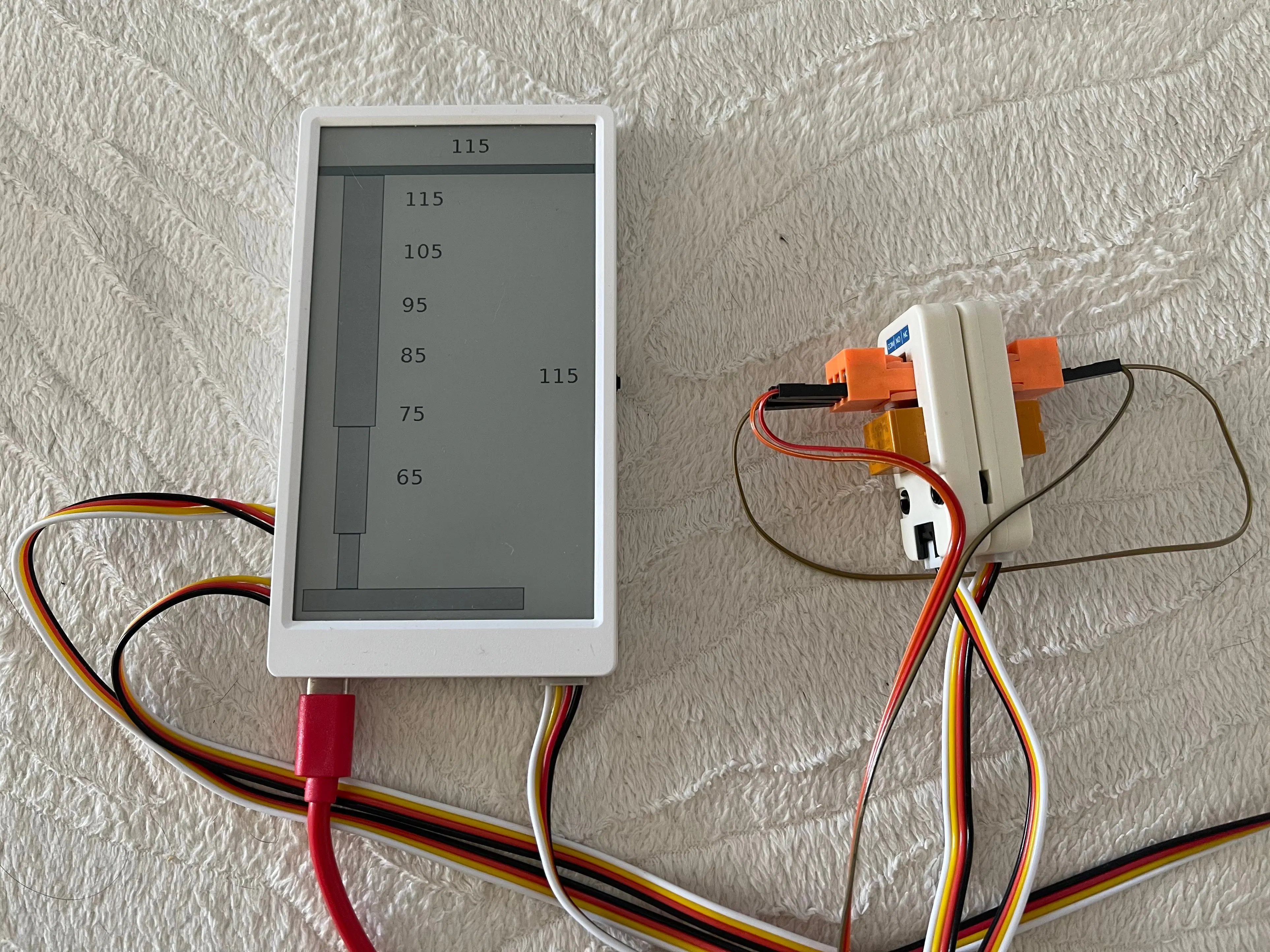## Things used in this project

### Hardware components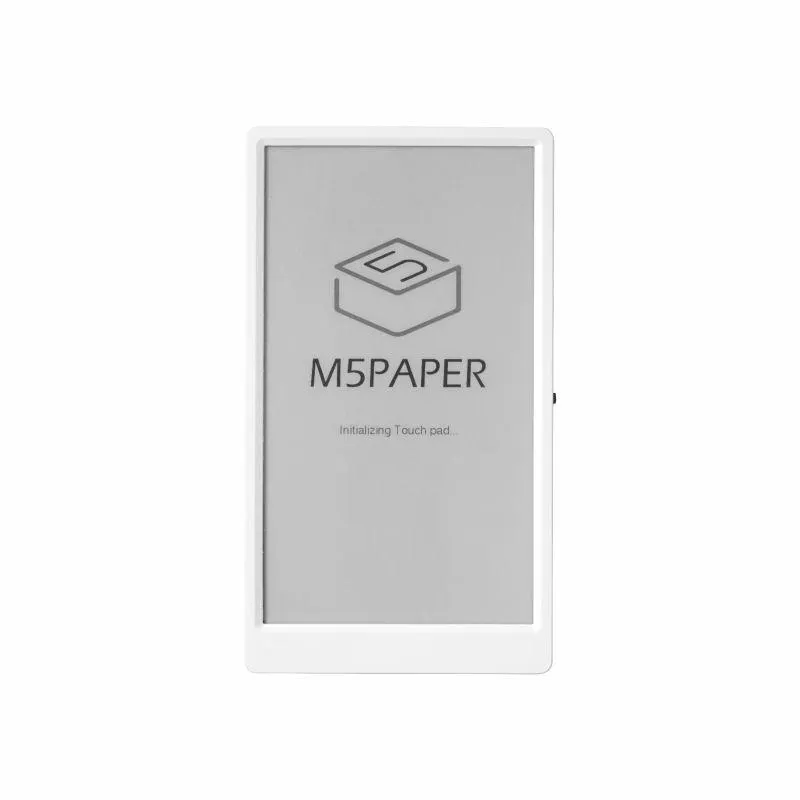M5Stack M5Paper ESP32 Development Kit (960X540, 4.7" eInk display, 235 ppi)
×1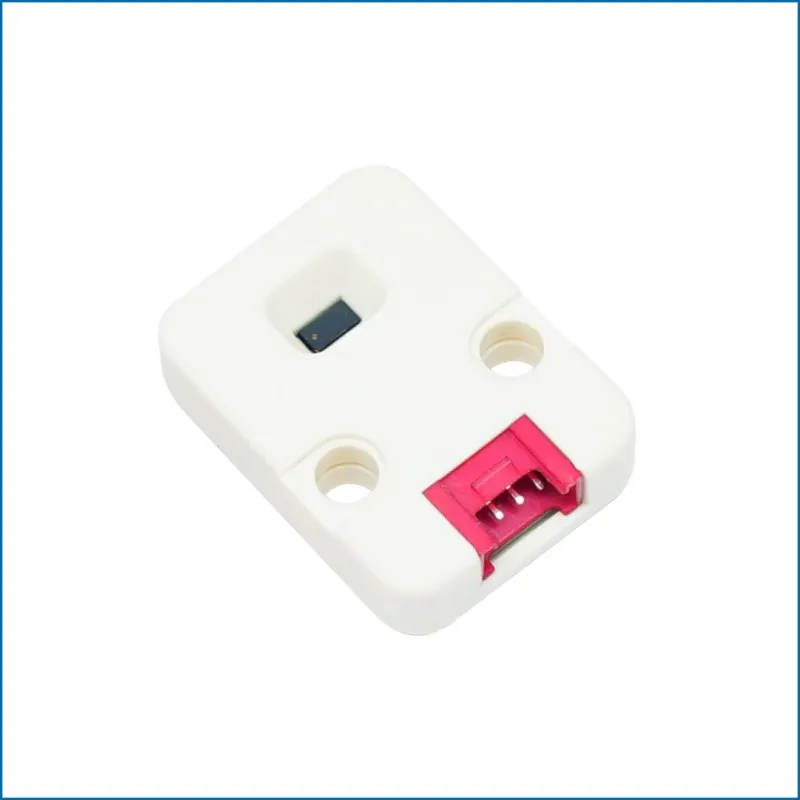M5Stack Time-of-Flight (ToF) VL53L0X Laser Ranging Unit (MCP4725/)
×1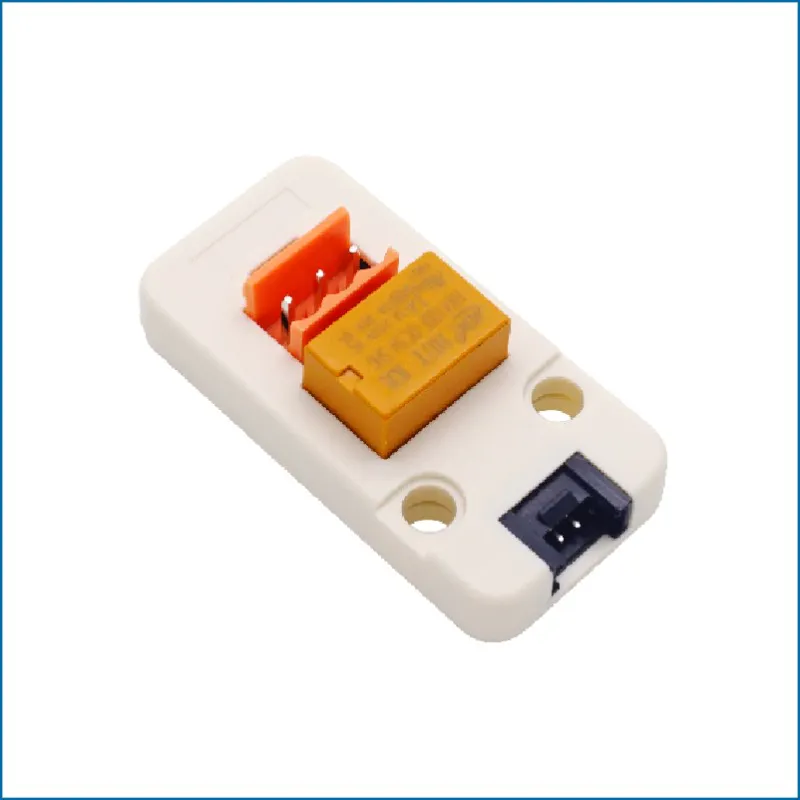M5Stack Mini 3A Relay Unit
×2
 IKEA BEKANT Desk sit/stand https://www.ikea.com/ch/en/cat/bekant-desk-underframes-18965/ 902.552.52 (Underframe sit/stand f table tp, el, white120x80 cm) https://www.ikea.com/ch/en/assembly_instructions/bekant-underframe-sit-stand-f-table-tp-el-white__AA-1006070-9_pub.pdf 302.552.50 (Underframe sit/stand f table tp, el120x80 cm) https://www.ikea.com/ch/en/assembly_instructions/bekant-underframe-sit-stand-f-table-tp-el__AA-1006070-9_pub.pdf
×1
 IKEA BEKANT Table top
×1
×1Jumper wires (generic) Make <=> Make (to attach to relays), Male <=> Female (to extend)
×1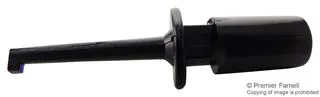Test Probe Connector, Hook Test Clamp Wire Hook Test Clip (usually for Logic Analyzer) but used to hook to original two button backplane circuit
×1

### Software apps and online services

 M5Stack UIFlow

### Hand tools and fabrication machines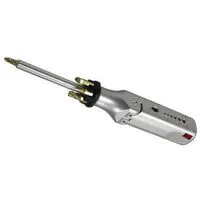Multitool, Screwdriver Used to break into the ABS enclosure of the original IKEA control (138684) also used for the 3.96 socket part of Relay M5Unit (Mini 3A Relay Unit) Part of iFixit's Pro Tech Toolkit
 Metal Spudger Used to break into the ABS enclosure of the original IKEA control (138684) Part of iFixit's Pro Tech Toolkit
 Halberd Spudger Used to break into the ABS enclosure of the original IKEA control (138684) Part of iFixit's Pro Tech ToolkitHot glue gun (generic)

## Schematics

### M5Stack UiFlow Blocky

Using M5Stack UiFlow v1.7.4 for M5stack M5Paper connected to two Relay M5Units (ports B + C) and one ToF M5Units (port A)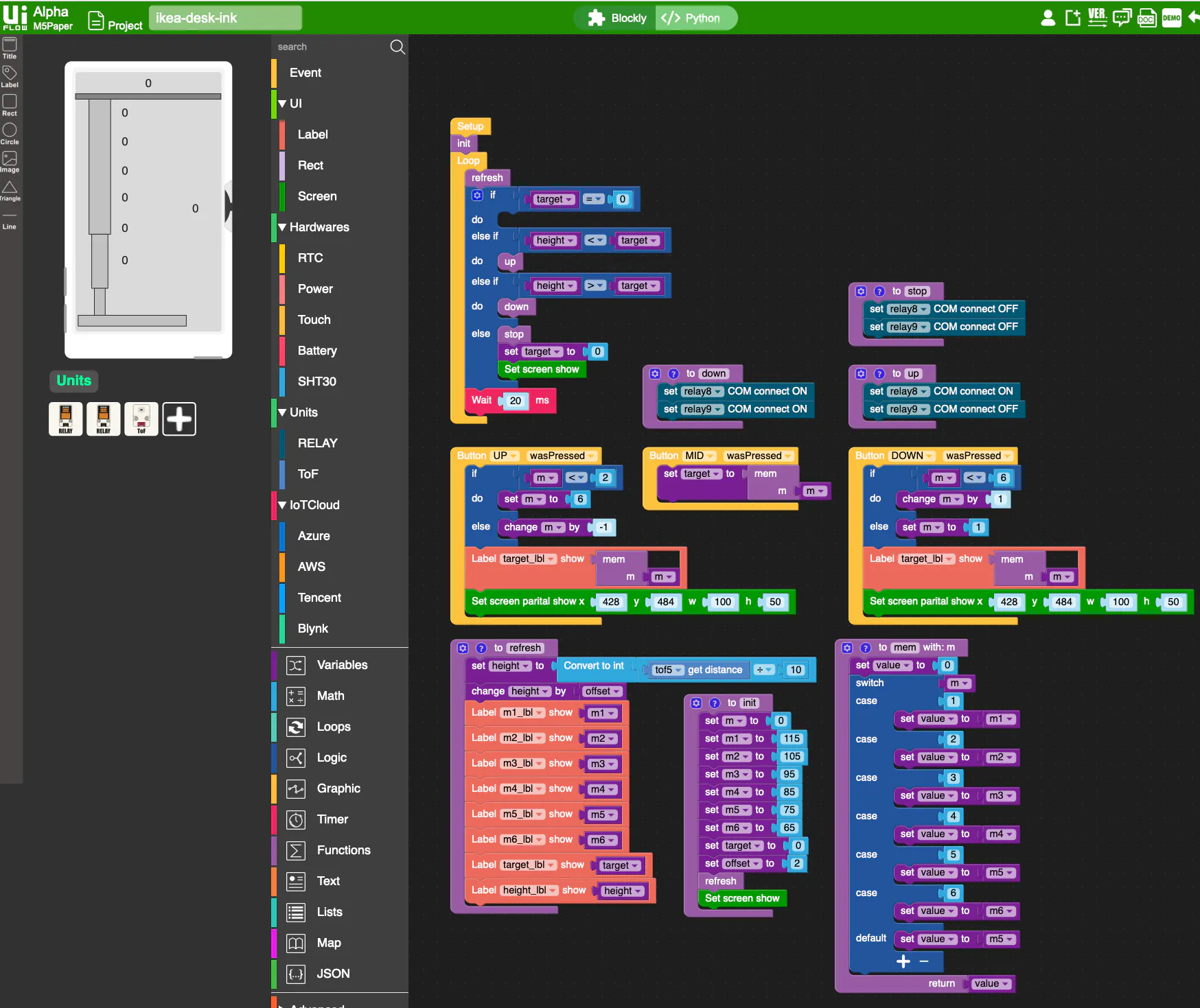### M5Stack UiFlow Blocky Code

Using M5Stack UiFlow v1.7.4 for M5stack M5Paper connected to two Relay M5Units (ports B + C) and one ToF M5Units (port A)

## Code

### M5Stack Python Code

Python
Using M5Stack UiFlow v1.7.4 for M5stack M5Paper connected to two Relay M5Units (ports B + C) and one ToF M5Units (port A)
```from m5stack import *
from m5ui import *
from uiflow import *
import time
import unit

setScreenColor(15)
relay8 = unit.get(unit.RELAY, unit.PORTB)
relay9 = unit.get(unit.RELAY, unit.PORTC)
tof5 = unit.get(unit.TOF, unit.PORTA)

m = None
target = None
height = None
value = None
m1 = None
offset = None
m2 = None
m3 = None
m4 = None
m5 = None
m6 = None

m1_lbl = M5TextBox(170, 133, "0", lcd.FONT_DejaVu40, 0, rotate=0)
m2_lbl = M5TextBox(170, 240, "0", lcd.FONT_DejaVu40, 0, rotate=0)
m3_lbl = M5TextBox(170, 347, "0", lcd.FONT_DejaVu40, 0, rotate=0)
m5_lbl = M5TextBox(170, 559, "0", lcd.FONT_DejaVu40, 0, rotate=0)
m6_lbl = M5TextBox(170, 679, "0", lcd.FONT_DejaVu40, 0, rotate=0)
target_lbl = M5TextBox(431, 487, "0", lcd.FONT_DejaVu40, 0, rotate=0)
m4_lbl = M5TextBox(170, 446, "0", lcd.FONT_DejaVu40, 0, rotate=0)
rectangle0 = M5Rect(10, 900, 400, 40, 13, 0)
rectangle1 = M5Rect(50, 100, 80, 500, 13, 0)
rectangle2 = M5Rect(60, 600, 60, 200, 13, 0)
rectangle3 = M5Rect(70, 800, 40, 100, 13, 0)
rectangle4 = M5Rect(0, 80, 538, 20, 8, 0)
height_lbl = M5TextBox(257, 24, "0", lcd.FONT_DejaVu40, 0, rotate=0)

from numbers import Number

# Describe this function...
def refresh():
global m, target, height, value, m1, offset, m2, m3, m4, m5, m6
height = int(((tof5.distance) / 10))
height = (height if isinstance(height, Number) else 0) + offset
m1_lbl.setText(str(m1))
m2_lbl.setText(str(m2))
m3_lbl.setText(str(m3))
m4_lbl.setText(str(m4))
m5_lbl.setText(str(m5))
m6_lbl.setText(str(m6))
target_lbl.setText(str(target))
height_lbl.setText(str(height))

# Describe this function...
def init():
global m, target, height, value, m1, offset, m2, m3, m4, m5, m6
m = 0
m1 = 115
m2 = 105
m3 = 95
m4 = 85
m5 = 75
m6 = 65
target = 0
offset = 2
refresh()
lcd.show()

# Describe this function...
def up():
global m, target, height, value, m1, offset, m2, m3, m4, m5, m6
relay8.on()
relay9.off()

# Describe this function...
def down():
global m, target, height, value, m1, offset, m2, m3, m4, m5, m6
relay8.on()
relay9.on()

# Describe this function...
def stop():
global m, target, height, value, m1, offset, m2, m3, m4, m5, m6
relay8.off()
relay9.off()

# Describe this function...
def mem(m):
global target, height, value, m1, offset, m2, m3, m4, m5, m6
value = 0
if m==1:
value = m1
elif m==2:
value = m2
elif m==3:
value = m3
elif m==4:
value = m4
elif m==5:
value = m5
elif m==6:
value = m6
else:
value = m5
return value

def buttonUP_wasPressed():
global target, height, m, value, m1, offset, m2, m3, m4, m5, m6
if m < 2:
m = 6
else:
m = (m if isinstance(m, Number) else 0) + -1
target_lbl.setText(str(mem(m)))
lcd.partial_show(428, 484, 100, 50)
pass
btnUP.wasPressed(buttonUP_wasPressed)

def buttonDOWN_wasPressed():
global target, height, m, value, m1, offset, m2, m3, m4, m5, m6
if m < 6:
m = (m if isinstance(m, Number) else 0) + 1
else:
m = 1
target_lbl.setText(str(mem(m)))
lcd.partial_show(428, 484, 100, 50)
pass
btnDOWN.wasPressed(buttonDOWN_wasPressed)

def buttonMID_wasPressed():
global target, height, m, value, m1, offset, m2, m3, m4, m5, m6
target = mem(m)
pass
btnMID.wasPressed(buttonMID_wasPressed)

init()
while True:
refresh()
if target == 0:
pass
elif height < target:
up()
elif height > target:
down()
else:
stop()
target = 0
lcd.show()
wait_ms(20)
wait_ms(2)
```

## Credits

### Baco

2 projects • 2 followers